1. Cube from sphere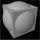What largest surface area (in cm2) can have a cube that was cut out of a sphere with radius 43 cm?
2. Chord distanceThe circle k (S, 6 cm), calculate the chord distance from the center circle S when the length of the chord is t = 10 cm.
3. Water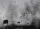In the garden with an area of 8 ares rain 40hl of water. To what heights leveled water?
4. Circle - simpleCalculate the area of a circle in dm2, if its circumference is 31.4 cm.
5. Hexagonal pyramidBase of the pyramid is a regular hexagon, which can be circumscribed in a circle with a radius of 1 meter. Calculate the volume of a pyramid 2.5 meters high.
6. Annulus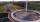Calculate the area of two circles annulus k1 (S, 3 cm) and k2 (S, 5 cm).
7. Rhombus A2p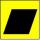Rhombus area is 13 cm2 and its height is 5cm long . Determine a perimeter of this rhombus.
8. Draw a trapezoidDraw a trapezoid if given a = 7 cm, b = 4 cm, c = 3.5 cm, diagonal AC = 5cm. Solve as a construction task.
9. Internal and external anglesCalculate the remaining internal and external angles of a triangle, if you know the internal angle γ (gamma) = 34 degrees and one external angle is 78 degrees and 40 '. Determine what kind of triangle it is from the size of its angles.
10. Grass seeds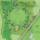How many kg of grass seed is needed to be sowing a circular field with a diameter of 120 meters when the 1 m2 should be used 0.8 grams seeds?
11. Roof tilesThe roof has a trapezoidal shape with bases of 15 m and 10 m, height of roof is 4 meters. How many tiles will need if on 1 m2 should be used 8 tiles?
12. SidewalkThe city park is a circular bed of flowers with a diameter of 8 meters, around it the whole length is 1 meter wide sidewalk . What is the sidewalk area?
13. Cuboid - simple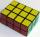Calculate the surface area and volume of a cuboid if a = 8 cm, b = 14 cm and c = 6 cm.
14. Tetrahedral prismCalculate surface and volume tetrahedral prism, which has a rhomboid-shaped base, and its dimensions are: a = 12 cm, b = 7 cm, ha = 6 cm and prism height h = 10 cm.
15. Right triangle ABCCalculate the perimeter and area of a right triangle ABC, if you know the length of legs 4 cm 5.5 cm and 6.8 cm is hypotenuse.
16. PrismThe volume of tetrahedral prism is 2.43 m3. Base of prism is a parallelogram in which a side 2,5dm and height ha = 18cm. Calculate the height of the prism.
17. Clock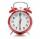What distance will pass end of 8 cm long hour hand for 15 minutes?
18. Triangular prismCalculate the surface area and volume of a triangular prism, base right triangle if a = 3 cm, b = 4 cm, c = 5 cm and height of prism h=12 cm.
19. Cuboids in cubeHow many cuboids with dimensions of 6 cm, 8 cm and 12 cm can fit into a cube with side 96 centimeters?
20. Stone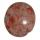When Peter threw stone in a box of water he discovered that the water level has risen by 6 cm. The box has a cuboid shape, the bottom has dimensions of 24 cm and 14 cm, height is 40 cm. What volume has a stone?

Do you have an interesting mathematical word problem that you can't solve it? Submit math problem, and we can try to solve it.

We will send a solution to your e-mail address. Solved examples are also published here. Please enter the e-mail correctly and check whether you don't have a full mailbox.

Please do not submit problems from current active competitions such as Mathematical Olympiad, correspondence seminars etc...